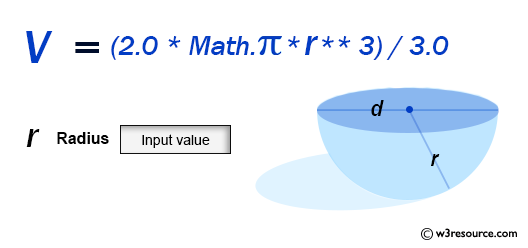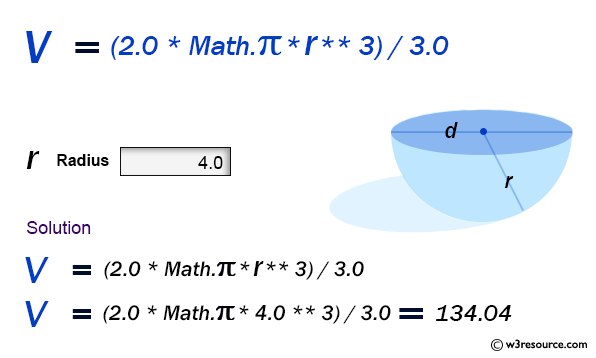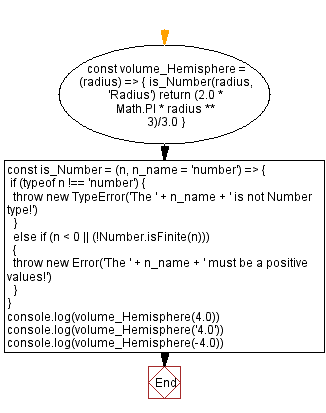# JavaScript: Volume of a Hemisphere

## JavaScript Math: Exercise-64 with Solution

Write a JavaScript program to calculate the volume of a Hemisphere.

Hemisphere refers to a half of a sphere:
A sphere is a geometrical object that is a three-dimensional analogue to a two-dimensional circle. A sphere is the set of points that are all at the same distance r from a given point in three-dimensional space. That given point is the centre of the sphere, and r is the sphere's radius. The earliest known mentions of spheres appear in the work of the ancient Greek mathematicians.

Sample Data:Sample Solution:

HTML Code:

``````<!DOCTYPE html>
<html>
<meta charset="utf-8">
<title>JavaScript function to Volume of a Hemisphere</title>
<body>

</body>
</html>
```
```

JavaScript Code:

``````const volume_Hemisphere = (radius) => {
return (2.0 * Math.PI * radius ** 3)/3.0
}
const is_Number = (n, n_name = 'number') => {
if (typeof n !== 'number') {
throw new TypeError('The ' + n_name + ' is not Number type!')
}
else if (n < 0 || (!Number.isFinite(n)))
{
throw new Error('The ' + n_name + ' must be a positive values!')
}
}
console.log(volume_Hemisphere(4.0))
console.log(volume_Hemisphere('4.0'))
console.log(volume_Hemisphere(-4.0))
```
```

Sample Output:

```134.0412865531645
-----------------------------------------------------
Uncaught TypeError: The Radius is not Number type!
at https://cdpn.io/cpe/boomboom/pen.js?key=pen.js-cdc3f8b5-64db-2243-c54a-9eb0b18e4e11:7
---------------------------------------------------------------------------------------
Uncaught Error: The Radius must be a positive values!
at https://cdpn.io/cpe/boomboom/pen.js?key=pen.js-1c4568d0-7438-2bbc-4808-62b08b7f3693:11
```

Flowchart:Live Demo:

See the Pen javascript-math-exercise-64 by w3resource (@w3resource) on CodePen.

Improve this sample solution and post your code through Disqus

Previous: Volume of a Sphere.
Next: Find Lucas number from index value

What is the difficulty level of this exercise?

Test your Programming skills with w3resource's quiz.

﻿